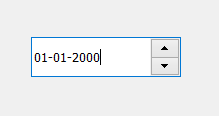# PyQt5 – QDateEdit

• Last Updated : 21 Jun, 2022

QDateEdit is the class that provide the date information, allowing user to input the date. It allows the user to edit dates by using the keyboard or the arrow keys to increase and decrease date. It is the widget majorly used for getting input dates. Below is how the date edit widget looks likeExample : A window having a QDateEdit and user can enter any date in it and when user press enter after changing the date, the entered date will get displayed in the label Below is the implementation

## Python3

 `# importing libraries``from` `PyQt5.QtWidgets ``import` `*``from` `PyQt5 ``import` `QtCore, QtGui``from` `PyQt5.QtGui ``import` `*``from` `PyQt5.QtCore ``import` `*``import` `sys`  `class` `Window(QMainWindow):` `    ``def` `__init__(``self``):``        ``super``().__init__()` `        ``# setting title``        ``self``.setWindowTitle("Python ")` `        ``# setting geometry``        ``self``.setGeometry(``100``, ``100``, ``500``, ``400``)` `        ``# calling method``        ``self``.UiComponents()` `        ``# showing all the widgets``        ``self``.show()` `    ``# method for components``    ``def` `UiComponents(``self``):` `        ``# creating a QDateEdit widget``        ``date ``=` `QDateEdit(``self``)` `        ``# setting geometry of the date edit``        ``date.setGeometry(``100``, ``100``, ``150``, ``40``)` `        ``# creating a label``        ``label ``=` `QLabel("GeeksforGeeks", ``self``)` `        ``# setting geometry``        ``label.setGeometry(``100``, ``150``, ``200``, ``60``)` `        ``# making label multiline``        ``label.setWordWrap(``True``)` `        ``# adding action to the date when enter key is pressed``        ``date.editingFinished.connect(``lambda``: date_method())` `        ``# method called by date edit``        ``def` `date_method():` `            ``# getting the date``            ``value ``=` `date.date()` `            ``# setting text to the label``            ``label.setText("Selected Date : " ``+` `str``(value))` `# create pyqt5 app``App ``=` `QApplication(sys.argv)` `# create the instance of our Window``window ``=` `Window()` `# start the app``sys.exit(App.``exec``())`

Output :

My Personal Notes arrow_drop_up